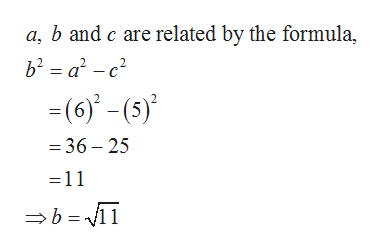# For Exercise, write the standard form of an equation of an ellipse subject to the given conditions. Vertices: (6, 0) and (−6, 0);Foci: (5, 0) and (−5, 0)

Question
26 views

For Exercise, write the standard form of an equation of an ellipse subject to the given conditions.

Vertices: (6, 0) and (−6, 0);

Foci: (5, 0) and (−5, 0)

check_circle

Step 1

Given:

Step 2

From the given information, the vertices are at distance 6 units from the center. Therefore, the value of a is 6.

Also, the foci are at distance 5 units from the center. Therefore, the value of c is 5.

And the center of the ellipse is the origin.

Now obtain the value of b as shown below.help_outlineImage Transcriptionclosea, b and c are related by the formula, b² = a² - c? =(6)' - (5)' = 36 – 25 =11 →b = 11 fullscreen
Step 3

Here the value of a is greater than b and the vertices are at x axis.

...

### Want to see the full answer?

See Solution

#### Want to see this answer and more?

Solutions are written by subject experts who are available 24/7. Questions are typically answered within 1 hour.*

See Solution
*Response times may vary by subject and question.
Tagged in

### Other